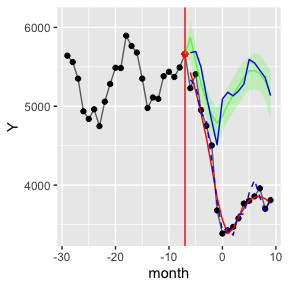Introduction

This vignette quickly outlines the primary method calls for conducting an analysis of an Interrupted Time Series using the simulation approach proposed in the companion paper.

We first cover a simple regression model, then show how to do smoothing, then seasonality. We also make a brief note about generating fake data for the purposes of conducting simulation studies.

Basic ITS analysis

We use the raw Mecklenberg data to illustrate the simITS package.

data(mecklenberg)
#> # A tibble: 6 x 10
#>   month  karr pbail pptrel  pror  pb4c avg_days_initial avg_t2d pstint7 pstint30
#>   <dbl> <dbl> <dbl>  <dbl> <dbl> <dbl>            <dbl>   <dbl>   <dbl>    <dbl>
#> 1   -29  2450 0.661 0.0347 0.304 0.634            11.4     193.   0.204   0.0820
#> 2   -28  2518 0.668 0.0365 0.295 0.636             9.96    188.   0.156   0.0564
#> 3   -27  2501 0.641 0.0424 0.317 0.628             9.68    191.   0.158   0.0636
#> 4   -26  2472 0.646 0.0376 0.316 0.649            13.9     183.   0.174   0.0781
#> 5   -25  2628 0.664 0.0438 0.293 0.655            12.8     184.   0.211   0.0799
#> 6   -24  2629 0.647 0.0312 0.322 0.697            10.1     193.   0.169   0.0677
meck = mutate( mecklenberg, pbail = 100 * pbail )
ggplot( meck, aes( x=month, y=pbail)) +
geom_rect(aes( ymin=-Inf, ymax=Inf, xmin=0.5, xmax=25, fill="lightgray"), col = "lightgray", alpha=0.25) +
scale_fill_identity(name = "", guide = "none", labels = c('Post Policy era')) +
geom_hline( yintercept = 0, col="black") +
geom_line( col="black", lty=1, lwd=0.5) +
geom_point() +
scale_x_continuous( breaks = c(-29,-24,-18,-12,-6,1,6,12,18,24)) +
coord_cartesian(xlim=c(-29.5,24.5), ylim=c(0,100), expand=FALSE) +
labs( title = " ", y = "Percent cases assigned bail", x = " " )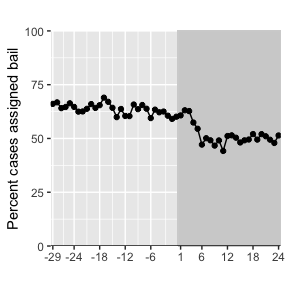To have autoregressive errors we use lagged outcomes. We can add lagged outcomes (and covariates) as so:

meck = add_lagged_covariates( meck, outcomename = "pbail", covariates=NULL )
sample_n( meck, 5 ) %>% arrange( month )
#> # A tibble: 5 x 11
#>   month  karr pbail pptrel  pror  pb4c avg_days_initial avg_t2d pstint7 pstint30
#>   <dbl> <dbl> <dbl>  <dbl> <dbl> <dbl>            <dbl>   <dbl>   <dbl>    <dbl>
#> 1   -19  2262  64.2 0.0385 0.320 0.757            11.5     189.   0.175   0.0637
#> 2    -4  1938  62.2 0.0289 0.349 0.686             8.94    216.   0.155   0.0599
#> 3     7  2061  50.1 0.0359 0.463 0.585             7.83    208.   0.154   0.0456
#> 4    18  1850  52.1 0.0319 0.448 0.588             7.75    161.   0.165   0.0551
#> 5    22  1993  49.4 0.0311 0.475 0.549             6.10    150.   0.127   0.0426
#> # … with 1 more variable: lag.outcome <dbl>

This package passes functions for fitting the model, and then uses these functions for doing the extrapolation. For the default, we use the package’s fit_model_default() which is a simple line (with lagged outcome as a covariate):

meck.pre = filter( meck, month <= 0 )
mod = fit_model_default( meck.pre, "pbail" )
summary( mod )
#>
#> Call:
#> stats::lm(formula = stats::as.formula(form), data = dat[-c(1),
#>     ])
#>
#> Residuals:
#>     Min      1Q  Median      3Q     Max
#> -3.7374 -1.6059 -0.2528  1.3190  4.6280
#>
#> Coefficients:
#>             Estimate Std. Error t value Pr(>|t|)
#> (Intercept) 44.99557   11.73346   3.835 0.000718 ***
#> month       -0.12320    0.05526  -2.229 0.034642 *
#> lag.outcome  0.26357    0.19033   1.385 0.177875
#> ---
#> Signif. codes:  0 '***' 0.001 '**' 0.01 '*' 0.05 '.' 0.1 ' ' 1
#>
#> Residual standard error: 2.094 on 26 degrees of freedom
#> Multiple R-squared:  0.3575, Adjusted R-squared:  0.3081
#> F-statistic: 7.234 on 2 and 26 DF,  p-value: 0.003178

To run the entire simulation and extrapolation as a call, we can directly do:

t0 = 0
envelope = process_outcome_model( "pbail", meck,
t0=t0, R = 100,
summarize = TRUE, smooth=FALSE )
sample_n( envelope, 5 ) %>% arrange( month )
#> # A tibble: 5 x 10
#>   month  Ymin  Ymax range    SE Ystar     Y Ysmooth Ysmooth1  Ybar
#>   <dbl> <dbl> <dbl> <dbl> <dbl> <dbl> <dbl> <lgl>   <lgl>    <dbl>
#> 1   -14  59.9  59.9   0    0     59.9  59.9 NA      NA        63.5
#> 2    -3  62.5  62.5   0    0     62.5  62.5 NA      NA        61.7
#> 3     6  53.3  64.9  11.5  2.98  60.3  47.1 NA      NA        60.2
#> 4    16  53.0  63.9  10.8  3.39  58.4  49.1 NA      NA        58.6
#> 5    18  51.3  63.8  12.5  3.20  58.0  52.1 NA      NA        58.2

And plotting our results:

ggplot( envelope, aes( month ) ) +
geom_line( aes( y=Y ), alpha = 0.6 ) +  # original data
geom_point( aes( y=Y ) ) +              # original data
geom_ribbon( aes( ymin=Ymin, ymax=Ymax ), alpha=0.2 ) +
geom_line( aes( y = Ystar ), col="darkgrey" ) +
geom_vline( xintercept = t0+0.5)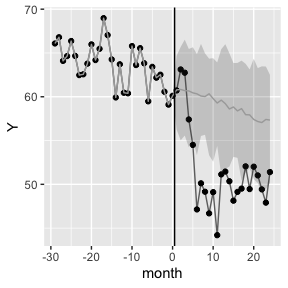We provide a nice utility function to generate these graphs:

make_envelope_graph(envelope, t0=t0)

Testing and Impact Intervals

We can aggregate impacts for several time points as follows. First call process_outcome_model() without summarizing:

predictions = process_outcome_model( "pbail", meck,
t0=t0, R = 100,
summarize = FALSE, smooth=FALSE )

Then use aggregate_simulation_results():

sstat = aggregate_simulation_results( orig.data = meck, outcomename = "pbail",
predictions = predictions, months = 1:18 )

quantile( sstat$t, c( 0.025, 0.975 )) #> 2.5% 97.5% #> 57.06750 62.40886 sstat$t.obs
#>  52.0368
sstat$t.obs - quantile( sstat$t, c( 0.025, 0.975 ))
#>       2.5%      97.5%
#>  -5.030692 -10.372056

Generating fake data

For simulation we also offer a fake data generator. It works like this:

dat = generate_fake_data( t_min=-60, t_max=18, t0 = 0 )
qplot( month, Y, data=dat, geom = c( "point","line") )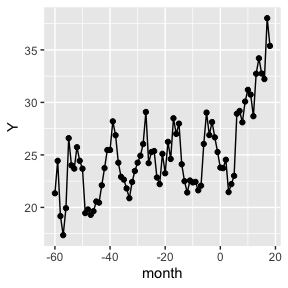Smoothing

Here we demonstrate summarizing and smoothing, using the fake data we just generated.

envelope = process_outcome_model( "Y", dat, t0=t0, R = 100,
summarize = TRUE, smooth=TRUE )
make_envelope_graph(envelope, t0 )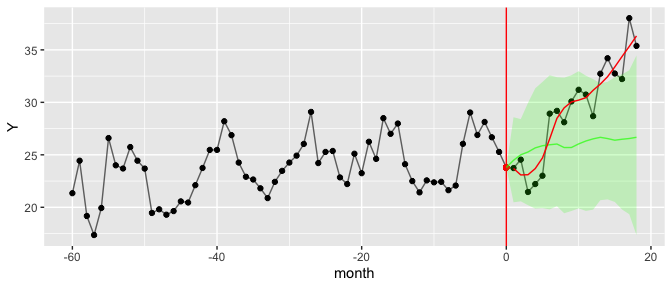We can smooth to different degrees using the smooth_k parameter:

alphas = c( 6, 11, 20, 100 )
preds = purrr::map( alphas, function( alpha ) {
pds = process_outcome_model( "Y", dat,
t0=t0, R = 20,
summarize = FALSE, smooth=TRUE,
smooth_k = alpha )
pds
} )
names( preds ) = alphas
preds = bind_rows( preds, .id="alpha_k" )
ggplot( filter( preds, month >= t0 ), aes( month, Ysmooth ) ) +
facet_wrap( ~ alpha_k ) +
geom_line( aes( group=Run, col=alpha_k ), alpha=0.5, na.rm=TRUE) +
geom_line( data=dat, aes( month, Y ), col="black", alpha=0.5 ) +
geom_vline( xintercept=t0, col="red" ) +
labs( x="month", y="proportion given bail")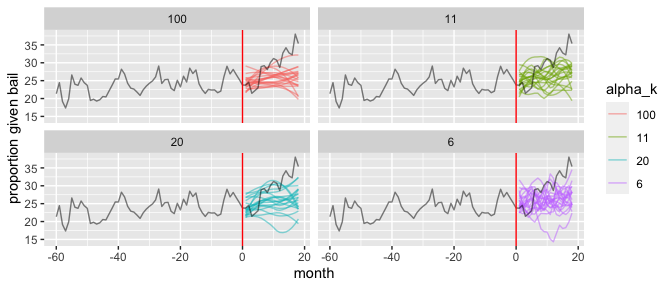Seasonality and covariates

A seasonality model on some fake data with a strong seasonality component is easy to fit. You just construct some code to fit the seasonality model via the make_fit_season_model() factory (you need to have the covariates pre-constructed in your data):

data( newjersey )
fit_season_model_qtemp =  make_fit_season_model( ~ temperature + Q2 + Q3 + Q4 )

envelope = process_outcome_model( "n.warrant", newjersey, t0=-7, R = 100,
summarize = TRUE, smooth=TRUE,
fit_model = fit_season_model_qtemp )
make_envelope_graph( envelope, t0=-7 )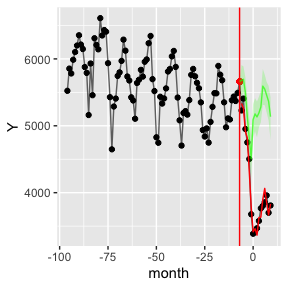Note how it will construct the lagged covariates automatically. The make_fit_season_model() method records what covariates are needed from the passed formula.

Smoothing and seasonality

We can smooth around a seasonality model either with a default smoother made from the specified seasonality model (as was done above) or, like the following, with a specified one of your choice:

smoother = make_model_smoother( fit_model = fit_season_model_sin, covariates = newjersey )
envelope_sin = process_outcome_model( "n.warrant", newjersey, t0=-7, R = 100,
summarize = TRUE, smooth=TRUE, smoother = smoother, smooth_k = 11,
fit_model = fit_season_model_qtemp )
envelope_sin$Ysmooth.base = envelope$Ysmooth
envelope_sin$Ysmooth1.base = envelope$Ysmooth1
make_envelope_graph( filter( envelope_sin, month > -30 ), t0=-7 ) +
geom_line( aes( y=Ysmooth.base ), col="blue", na.rm=TRUE ) +
geom_line( aes( y=Ysmooth1.base ), col="blue", lty=2, na.rm=TRUE )